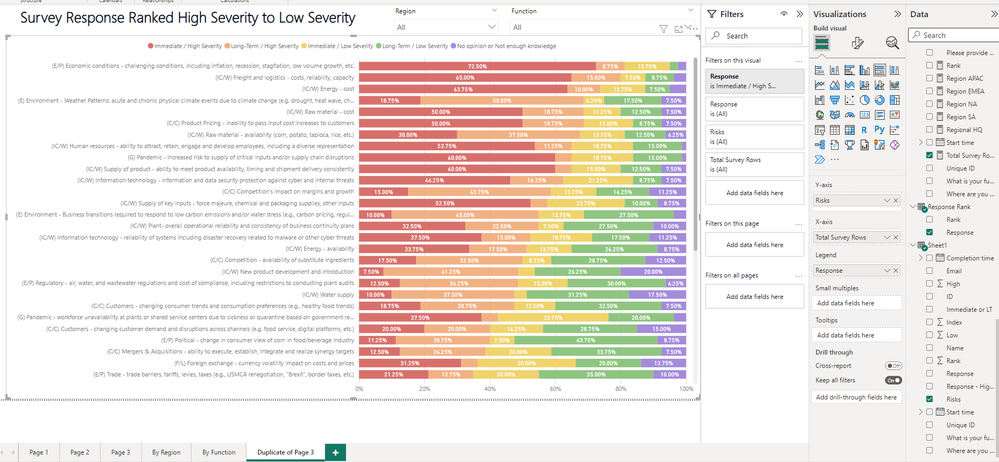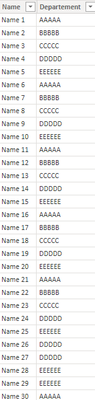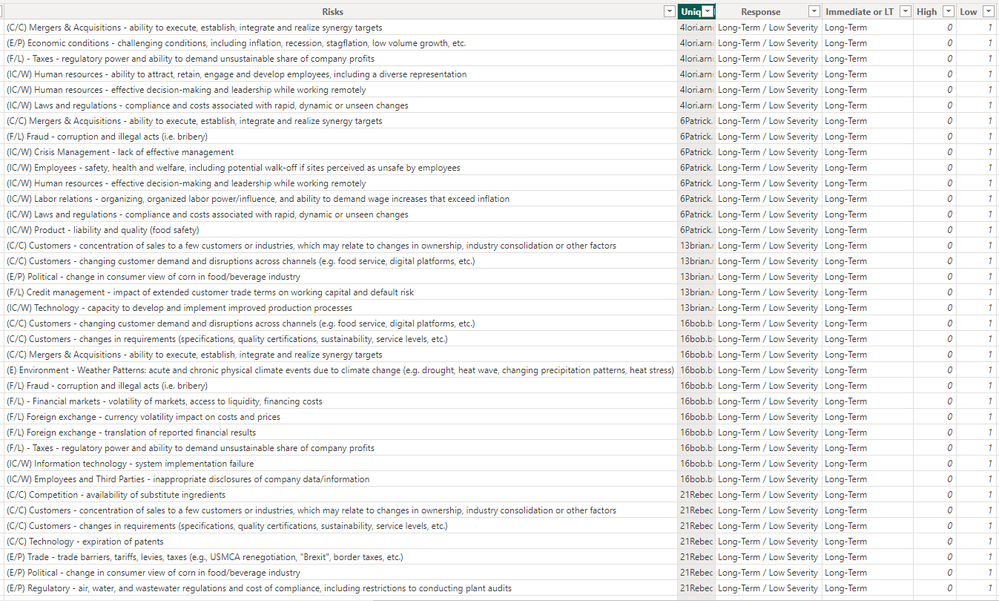cancel
Showing results for
Did you mean:Frequent Visitor

## Dynamic Table Ranking

I have a 100% stacked bar chart that is filtered by questions (Column name Risks) and the responses. In the screenshot below the measure is the total number of survey responses. In excel I built a pivot table to count total of responses that are "High Severity" for the responses. I added that cloumn into Sheet 1 named "Rank", I then sorted the responses by that rank. However, I would also like to filter this table by the Region and Funtion of the respondees. When I add the filter since they are sorted, it will not update the risks rank. What measure would I need to dynamically rank the questions (Column name Risks) to always show the highest amount of "high severity" responses depending on the filters?

Please let me know if anymore information is needed to solve this problem.1 ACCEPTED SOLUTIONCommunity Support

You can use FILTER() and CONTAINSSTRING() function.

e.g

``Measure = RANKX(ALLSELECTED('Table'[Type]),CALCULATE(COUNTROWS(FILTER('Table',CONTAINSSTRING([Response],"High")))),,ASC,Dense)``

You can refer to the following link to know more about the functions

CONTAINSSTRING function (DAX) - DAX | Microsoft Learn

FILTER function (DAX) - DAX | Microsoft Learn

Best Regards!

Yolo Zhu

If this post helps, then please consider Accept it as the solution to help the other members find it more quickly.

3 REPLIES 3Community Support

You can create a rank measure that use the rankx() function. then put the measure to the tooltip.

e.g Sample dataMeasure

``Measure = RANKX(ALLSELECTED(Austritt[Departement]),CALCULATE(COUNTROWS(Austritt)),,ASC,Skip)``

Then put the measure to the visual tooltips, then select sort by measureThen you can sort the visual by the dynamic rank.

Best Regards!

Yolo Zhu

If this post helps, then please consider Accept it as the solution to help the other members find it more quickly.Frequent Visitor

Hello-

I am still struggling with the formula above. When I use this it is still not ranking correctly. Example below.I believe the problem is because I need it to sort by a specific column. I created a column "High" that has 1 one listed next to the risk if it is considered a high severity risk. I need a formula that will count the total number of high severity in column "High". This would needed to be listed in the rank formula so it is ranking the risk by total number of High severity (From Column "high"). I believe the rankx formula is what is needed as it needs to dynamically update highest risk when filter by specifc region/function.Community Support

You can use FILTER() and CONTAINSSTRING() function.

e.g

``Measure = RANKX(ALLSELECTED('Table'[Type]),CALCULATE(COUNTROWS(FILTER('Table',CONTAINSSTRING([Response],"High")))),,ASC,Dense)``

You can refer to the following link to know more about the functions

CONTAINSSTRING function (DAX) - DAX | Microsoft Learn

FILTER function (DAX) - DAX | Microsoft Learn

Best Regards!

Yolo Zhu

If this post helps, then please consider Accept it as the solution to help the other members find it more quickly.